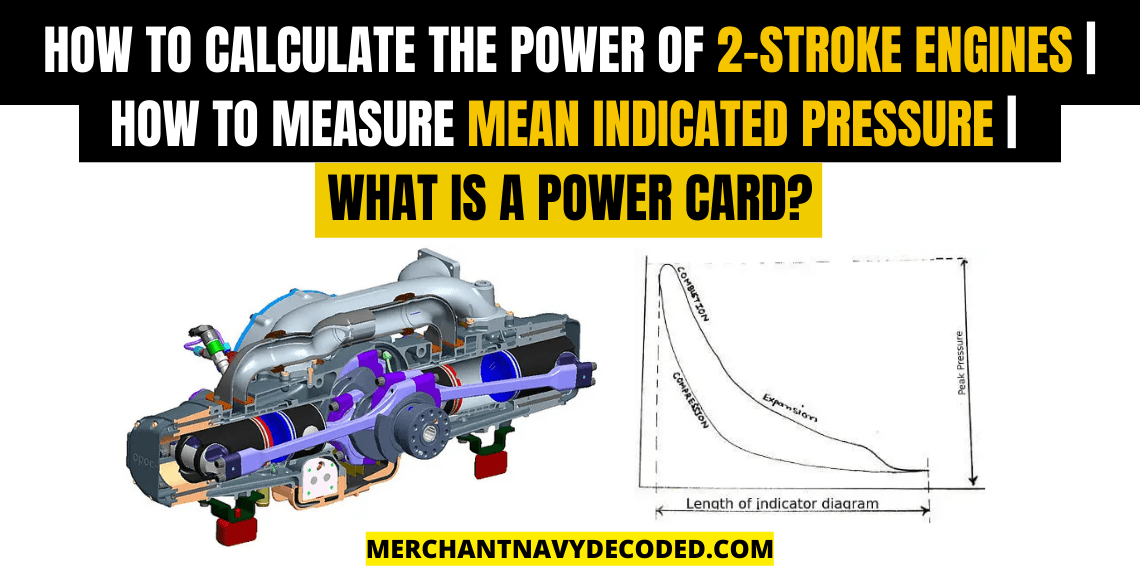## How to calculate the power of 2-stroke engines |How to measure Mean Indicated Pressure |What is a power card?

Last updated on May 9th, 2023 at 06:01 am

## How is the power of a two stroke engine calculated?

There are three ways to measure the power of a two stroke engine:

1. By Power Card: This is the old method of calculating power, where with the help of the power card diagram and planimeter we calculate the indicated horsepower generated by that particular unit.
1. By Digital Pressure Indicator: Unlike the power card method, which is tedious and requires skill set, in this case the company provides a digital pressure indicator which is mounted on the indicator cock and the DPI measures all the readings and displays on its screen as well as record it.

3. Intelligent Combustion Monitoring: The new generation engines are continuously monitored by ICM, which measures the real time in-cylinder pressure in all engine cylinders. Today in ME engines we are using this technique.

In all these three ways mentioned above they all use the same concept that is calculating the pressure in a cylinder at various angles, plotting it on a graph, which in turn is called power card. The only difference is that in the first method we calculate the power ourselves and in the other two it is calculated by the machine.

So let us calculate the power of a two stroke engine using Power card and Planimeter.The power of a two stroke engine is calculated today using digital meters with the help of a power card and a planimeter.

## Equation to find power generated by an engine

What is power?

Power = Work Done ÷ Time

What is work done?

Work Done= Force x Displacement

What is force?

Force = Pressure x Area

Power = (Pressure x Area x Displacement) ÷ Time

The pressure mentioned over here is the mean effective pressure which is the average pressure acting on a piston during the different portions of its cycle. As we know the pressure acting on the piston throughout the cycle varies, so we have to calculate the average pressure acting on the piston using the power card.

Also note, when we consider the power produced by an engine we must also consider the number of revolutions per second that the engine is running at, since power is produced during each revolution.

So power produced by a 2 stroke engine can be expressed as,

Power = Mean Indicated Pressure x Area x Displacement x Number of Revolutions per second

Area in this case is the area of the cylinder liner which is (𝝅/4), where D is the diameter of the cylinder liner.

Displacement in this case is the stroke length

## How to calculate mean pressure of a marine engine?

Mean Indicated Pressure = Area of Curve ÷ Length of Curve

The area of the curve is measured using the planimeter and when this area is divided by the length of the diagram and multiplied with the spring constant we get the mean indicated pressure.

Once we have calculated the mean indicated pressure of that particular unit then calculating indicated horsepower is a cakewalk as we know that,

IHP= Pm x Area x Stroke Length x n

2 1 vote
Article Rating
Subscribe
Notify of1 Comment
Inline Feedbacks